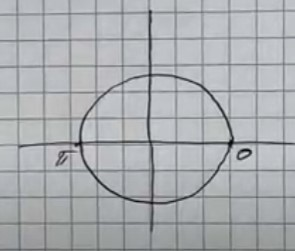# Find all values of x in the interval [0, 2pi] that satisfy the equation: sin x=tan xveneciasp 2022-07-13 Answered
Find all values of x in the interval $\left[0,2\pi \right]$ that satisfy the equation:
$\mathrm{sin}x=\mathrm{tan}x$
You can still ask an expert for help

• Live experts 24/7
• Questions are typically answered in as fast as 30 minutes
• Personalized clear answers

Solve your problem for the price of one coffee

• Math expert for every subject
• Pay only if we can solve itJayvion Mclaughlin
$\mathrm{sin}x=\mathrm{tan}x$
$\mathrm{sin}x=\frac{\mathrm{sin}x}{\mathrm{cos}x}$
$\mathrm{sin}x-\frac{\mathrm{sin}x}{\mathrm{cos}x}=0$
$\mathrm{sin}x\left(1-\frac{1}{\mathrm{cos}x}\right)=0$
$1-\frac{1}{\mathrm{cos}x}=0$
$1=\frac{1}{\mathrm{cos}x}$
$\mathrm{cos}x=1$
$\mathrm{sin}x=0;$
$x=0;\pi ;2\pi$###### Not exactly what you’re looking for?Jeffrey Jordon